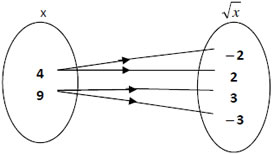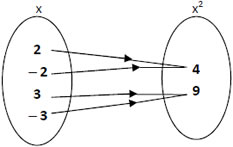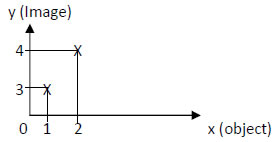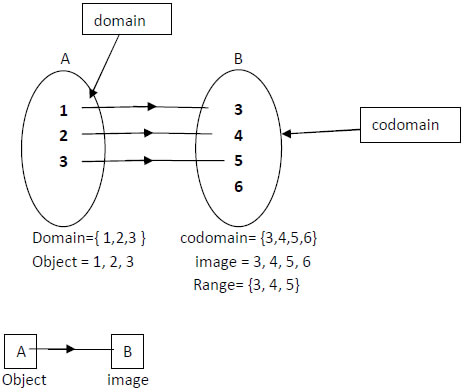Visitors Online: 77 | Thursday 14th November 2019

CBSE Mathematics Class XI

CBSE Guess > eBooks > Class XI > Mathematics

Mathematics - CBSE CLASS XI

Mathematics

CHAPTER 1- FUNCTIONS

1.1 RELATIONS
1.1.1 Representing Relations

1- By Arrow Diagram

 One to one relation One to many relationMany to one relation Many to many relation2- By Ordered Pair

(1, 3), (2, 4), (3, 5)

(1, 4), ( −2, 4), (3, 9), ( −3, 9)

3. By GraphSet A is called domain of the relation and set B is the codomain.
Each of the elements in the domain (Set A) is an object. So 1, 2 and 3 are called object.
Each of the elements in the codomain (Set B) is an image. So 3, 4, 5 and 6 are called image.
The set that contains all the images that are matched is the range.CBSE Mathematics Class XI ( By Mr. Ayaz Khan )
Email Id : khan7190@gmail.com